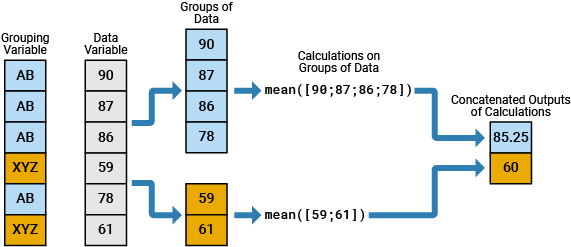# findgroups

## 语法

``G = findgroups(A)``
``G = findgroups(A1,...,AN)``
``[G,ID] = findgroups(A)``
``[G,ID1,...,IDN] = findgroups(A1,...,AN)``
``G = findgroups(T)``
``[G,TID] = findgroups(T)``

## 说明

``G = findgroups(A)` 返回 `G`，它是从分组变量 `A` 生成的组编号的向量。输出参数 `G` 包含从 1 到 `N` 的整数值，指示 `A` 中 `N` 个唯一值的 `N` 个不同组。例如，如果 `A` 是 `{'b','a','a','b'}`，则 `findgroups` 返回 `G` 为 `[2 1 1 2]`。您可以使用 `G` 拆分来自其他变量的数据组。使用 `G` 作为 拆分-应用-合并工作流 中 `splitapply` 的输入参数。`findgroups` 将 `A` 中的空字符向量和 `NaN`、`NaT` 以及未定义的分类值视为缺失值，并返回 `NaN` 作为 `G` 的对应元素。`

``G = findgroups(A1,...,AN)` 根据 `A1,...,AN` 生成组编号。`findgroups` 函数将组定义为 `A1,...,AN` 中唯一的值组合。例如，如果 `A1` 是 `{'a','a','b','b'}` 且 `A2` 是 `[0 1 0 0]`，则 `findgroups(A1,A2)` 返回 `G` 为 `[1 2 3 3]`，因为组合 `'b' 0` 出现两次。`

``[G,ID] = findgroups(A)` 还会在 `ID` 中返回每个组的唯一值。例如，如果 `A` 是 `{'b','a','a','b'}`，则 `findgroups` 返回 `G` 为 `[2 1 1 2]`，并返回 `ID` 为 `{'a','b'}`。参数 `A` 和 `ID` 具有相同的数据类型，但不需要具有相同的大小。`

``[G,ID1,...,IDN] = findgroups(A1,...,AN)` 还返回 `ID1,...,IDN` 中每个组的唯一值。`ID1,...,IDN` 中的值定义各组。例如，如果 `A1` 是 `{'a','a','b','b'}` 且 `A2` 是 `[0 1 0 0]`，则 `findgroups(A1,A2)` 返回 `G` 为 `[1 2 3 3]`，并返回 `ID1` 和 `ID2` 为 `{'a','a','b'}` 和 `[0 1 0]`。`

``G = findgroups(T)` 返回 `G`，它是从表 `T` 中的变量生成的组编号的向量。`findgroups` 函数将 `T` 中的所有变量视为分组变量。`

``[G,TID] = findgroups(T)` 还返回一个表 `TID`，其中包含每个组的唯一值。`TID` 包含 `T` 的所有变量中唯一的值组合。`T` 和 `TID` 中的变量具有相同的名称，但这些表不需要具有相同的行数。`

## 示例

```load patients whos Gender Height```
``` Name Size Bytes Class Attributes Gender 100x1 11412 cell Height 100x1 800 double ```

`G = findgroups(Gender);`

`Gender``G` 的前五个元素进行比较。其中 `Gender` 包含 `'Female'``G` 包含 `1`。其中 `Gender` 包含 `'Male'``G` 包含 `2`

`Gender(1:5)`
```ans = 5x1 cell {'Male' } {'Male' } {'Female'} {'Female'} {'Female'} ```
`G(1:5)`
```ans = 5×1 2 2 1 1 1 ```

`splitapply(@mean,Height,G)`
```ans = 2×1 65.1509 69.2340 ```

```load patients whos Systolic Diastolic Gender Smoker```
``` Name Size Bytes Class Attributes Diastolic 100x1 800 double Gender 100x1 11412 cell Smoker 100x1 100 logical Systolic 100x1 800 double ```

```G = findgroups(Smoker,Gender); G(1:10)```
```ans = 10×1 4 2 1 1 1 1 3 2 2 1 ```

```meanSystolic = splitapply(@mean,Systolic,G); meanDiastolic = splitapply(@mean,Diastolic,G); mBP = [meanSystolic,meanDiastolic]```
```mBP = 4×2 119.4250 79.0500 119.3462 79.8846 129.0000 89.2308 129.5714 90.3333 ```

```load patients whos Gender Height```
``` Name Size Bytes Class Attributes Gender 100x1 11412 cell Height 100x1 800 double ```

`[G,gender] = findgroups(Gender);`

```medianHeight = splitapply(@median,Height,G); T = table(gender,medianHeight)```
```T=2×2 table gender medianHeight __________ ____________ {'Female'} 65 {'Male' } 69 ```

```load patients whos Systolic Diastolic Gender Smoker```
``` Name Size Bytes Class Attributes Diastolic 100x1 800 double Gender 100x1 11412 cell Smoker 100x1 100 logical Systolic 100x1 800 double ```

```[G,gender,smoker] = findgroups(Gender,Smoker); meanSystolic = splitapply(@mean,Systolic,G); meanDiastolic = splitapply(@mean,Diastolic,G);```

`T = table(gender,smoker,meanSystolic,meanDiastolic)`
```T=4×4 table gender smoker meanSystolic meanDiastolic __________ ______ ____________ _____________ {'Female'} false 119.42 79.05 {'Female'} true 129 89.231 {'Male' } false 119.35 79.885 {'Male' } true 129.57 90.333 ```

```load patients T = table(Gender,Smoker); T(1:5,:)```
```ans=5×2 table Gender Smoker __________ ______ {'Male' } true {'Male' } false {'Female'} false {'Female'} false {'Female'} false ```

`G = findgroups(T);`

```meanSystolic = splitapply(@mean,Systolic,G); meanDiastolic = splitapply(@mean,Diastolic,G); mBP = [meanSystolic,meanDiastolic]```
```mBP = 4×2 119.4250 79.0500 129.0000 89.2308 119.3462 79.8846 129.5714 90.3333 ```

```load patients T = table(Gender,Smoker);```

```[G,TID] = findgroups(T); TID```
```TID=4×2 table Gender Smoker __________ ______ {'Female'} false {'Female'} true {'Male' } false {'Male' } true ```

```TID.meanSystolic = splitapply(@mean,Systolic,G); TID.meanDiastolic = splitapply(@mean,Diastolic,G)```
```TID=4×4 table Gender Smoker meanSystolic meanDiastolic __________ ______ ____________ _____________ {'Female'} false 119.42 79.05 {'Female'} true 129 89.231 {'Male' } false 119.35 79.885 {'Male' } true 129.57 90.333 ```

## 输出参数

• 如果分组变量为向量，则 `G` 和分组变量都具有相同的大小。

• 如果分组变量在一个表中，则 `G` 的长度等于该表的行数。

## 详细信息

### 拆分-应用-合并工作流